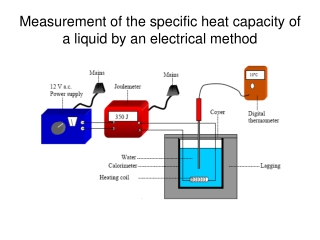DownloadDownload PresentationMeasurement of the specific heat capacity of a liquid by an electrical method

# Measurement of the specific heat capacity of a liquid by an electrical method

Download Presentation## Measurement of the specific heat capacity of a liquid by an electrical method

- - - - - - - - - - - - - - - - - - - - - - - - - - - E N D - - - - - - - - - - - - - - - - - - - - - - - - - - -
##### Presentation Transcript

1. Measurement of the specific heat capacity of a liquid by an electrical method

2. Procedure 1. Find the mass of the calorimeter mcal. 2. Find the mass of the calorimeter plus the water m1. Hence the mass of the water mw is m1 – mcal. 3. Set up the apparatus as shown. Record the initial temperature θ1. 4. Switch on the power supply and allow current to flow until a temperature rise of 10 °C has been achieved. 5. Switch off the power supply, stir the water well and record the highest temperature θ2. Hence the rise in temperature Δθ is θ2 – θ1. 6. Record the final joulemeter reading Q.

3. Results • Mass of the calorimeter mcal = • Mass of the calorimeter plus the water m1 = • Mass of the water mw = m1 – mcal = • Initial temperature of water θ1 = • Final temperature θ2 = • Rise in temperature Δθ = θ2 – θ1 = • Final joulemeter reading Q = Electrical energy supplied = energy gained by water + energy gained by calorimeter Q = mwcwθΔ + mcalccalθΔ.

4. Precautions Use a thermometer with a low specific heat capacity. Repeat and find an average.